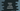# How to write a simple stopwatch in python## How to write a simple stopwatch in python:

In this post, we will learn how to write a simple stopwatch in python. It is a simple stopwatch and it will show the time spend on user inputs.

### time module:

For dealing with time, python provides a module called time. We can use this module to get the time spend in the stopwatch program. We will use the time() function defined in this module. This function returns the epoch time or the number of seconds passed since epoch.

Our program will record this epoch time when the stopwatch starts and when it ends. The difference is the total time spend in seconds. If we convert the seconds to any human-readable format, that is the time recorded by the stopwatch.

### Python program:

Below is the complete python program:

``````import time

def print_time(total_seconds):
total_mins = total_seconds / 60
seconds = int(total_seconds % 60)
hours = int(total_mins / 60)
mins = int(total_mins % 60)
print('Time spend: {}h:{}m:{}s'.format(hours, mins, seconds))

input('Press any key to start: ')
start_time = time.time()

print('counting time...')

input('Press any key to stop: ')
stop_time = time.time()

print_time(stop_time - start_time)``````

Here, The stopwatch is recording the starting time once the user presses any key. It reads the time, i.e. epoch time by using time.time() and stores that in the variable start_time. The stopwatch stops when the user presses any key again. It records the time again and keeps it in the stop_time variable. print_time method is used to print the time spent in hour-minute-seconds format. It gets the total number of seconds spent as the parameter and converts the value to hour: minute: seconds. hours, mins, and seconds variables hold the values of hours, minutes, and seconds for the total seconds. Finally, it prints the converted value.

If you run this program, it will print output as like below:

``````Press any key to start:
counting time...
Press any key to stop:
Time spend: 0h:4m:19s``````